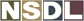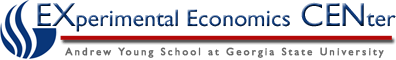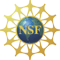Students / Subjects# Price Elasticity

Price Elasticity of demand or supply gives economists and business owners exact measures of the quantity response to a change in price. In other words, the measure tells us exactly how much the quantity supplied or demanded changes as a result of a change in the price. For this reason, price elasticity is the most commonly used elasticity concept.

The price elasticity of demand measures the responsiveness of quantity demanded due to a change in price.

E=change in quantity demandedChange in Price

The price elasticity of supply measures the responsiveness of quantity supplied due to a change in price.

E=change in quantity suppliedChange in Price

Example:

Suppose the quantity supplied of chopsticks increases by 4% and the price increases by 6%. Calculate the Elasticity of Supply as follows...

E= 4/6 = .67

Example:

Suppose the price of Bob Dylan concert tickets increase 15% and the quantity demanded decreases by 1.5%. Calculate the Elasticity of Demand as...

E= -1.5/15 =-.10Notice the decrease in quantity demanded is -1.5% which is a negative number. However, economists talk about price elasticities in terms of positive numbers. (Recall that the absolute value-1.5%is simply positive 1.5%).

It is important to note that elasticity is not the same as slope. However, the steeper the demand or supply curve, the more inelastic the curve is.

Characterizing Elasticity:

Elastic (E>1). We say that a good is (price) elastic when we find the elasticity to be greater than 1. Quantity demanded or supplied responds quite a bit to a change in price.

-We typically look at goods that have elastic demand or supply curves as ones with many substitutes. Typically, we see Coca Cola and Pepsi as substitutes**. For example, if the price of Pepsi were to increase, people are more likely to purchase Coca Cola. We can say that consumers are basically indifferent between the two products; this makes consumers sensitive to the price.

Inelastic (E<1). We say that a good is (price) inelastic if we find the elasticity to be less than 1. Quantity supplied or demanded is not very responsive to a change in price.

-Oil is an example of an inelastic good in the United States. Americans depend on their cars to get to work. Further, the majority of Americans do not have access to commute alternatives such as electric rail (few substitutes for oil). Therefore, if the price of oil increases, the demand for gasoline will not be impacted much. This is particularly true in the short run.

Unit Elastic (E=1). A good is unit elastic if we calculate the elasticity to be equal to 1.

Perfectly Elastic (E=). The quantity supplied or demanded responds a great deal to a change in price. This is characterized by a horizontal line on a graph.

-A perfectly elastic good implies easily accessible substitutes and perfect information. In other words, if the price of a increases, no units will be bought. For example, consider two nearly identical brands of salsa. If the price of one the goods increases (and they are perfectly elastic), no units of this good will be bought. You will not find a real example of this in the "real world".

Perfectly Inelastic (E=0). The quantity supplied or demanded does not change at all when there is a change in price. This is characterized by a vertical line on a graph.

-For an example, we can think of a diabetic's insulin as a perfectly inelastic. Presumably, because a diabetic depends on insulin to keep him or herself alive, the insulin will be bought no matter how much it costs.

Elasticity: The Individual vs. "in general" .